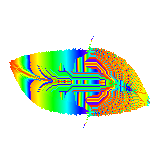# Dynamical Systems:tutorialDynamical systems are models containing the rules describing the way some quantity undergoes a change through time. These rules only involve the current value of the quantity.For example the motion of a pendulum can be modelled as a dynamical system. A dynamical system is called discrete if time is measured in discrete steps; these are modeled as recursive relations like the logistic map:
`x[n+1]=c*x[n]*(1-x[n])`
where n denotes the discrete time steps and x is the quantity changing over time. If time is measured continuously, the resulting continuous dynamical systems are expressed as ordinary differential equations, for instance
`dx/dt = c*x*(1-x)`
where x is the quantity that changes with time t. Here we will consider only discrete dynamical systems.
You can think about dynamical systems
• (if you are a computer graphics programmer): as a way to produce striking images.
• (if you are a mathematician):as iterated maps of the plane or algorithms that solve numerically systems of differential equations.
• (if you are a physicist):as a way to describe the behaviour of real-world things such as planetary motion,fluid flow,the weather,the Stock Exchange,etc

This may seem very complex, but from the point of view of the computer graphics programmer, it is very simple. In the end you have a formula that for a system described by two variables,like a pendulum in the plane, is a planar discrete system of the form:

```    x = f(x,y)
y = g(x,y)
```
When this formula is iterated starting from some initial point x0,y0 you are in fact solving numerically a differential equation, describing the behavior in time, of some physical system, like a pendulum, where x and y may be the space s along the trajectory and the speed v.
BACK to Dynamical Systems Data BaseMaintained by Giuseppe Zito : Giuseppe.Zito@cern.ch